# Sample JEE(MAIN) Physics Mock Test

## 30 Questions MCQ Test JEE Main Mock Test Series 2021 & Previous Year Papers | Sample JEE(MAIN) Physics Mock Test

Description
This mock test of Sample JEE(MAIN) Physics Mock Test for JEE helps you for every JEE entrance exam. This contains 30 Multiple Choice Questions for JEE Sample JEE(MAIN) Physics Mock Test (mcq) to study with solutions a complete question bank. The solved questions answers in this Sample JEE(MAIN) Physics Mock Test quiz give you a good mix of easy questions and tough questions. JEE students definitely take this Sample JEE(MAIN) Physics Mock Test exercise for a better result in the exam. You can find other Sample JEE(MAIN) Physics Mock Test extra questions, long questions & short questions for JEE on EduRev as well by searching above.
QUESTION: 1

### Number of electrons emitted by a surface exposed to light is directly proportional to

Solution:

Key Idea Number of photoelectrons emitted proportional to intensity of incident light.

For a given metal, rate of emission of photo electrons i.e., photocurrent is directly proportional to the intensity of incident radiation for a given light i.e., a bright light always gives more photocurrent than a dim one for a given frequency.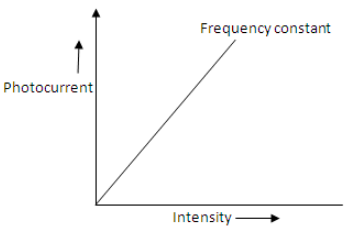Intensity here means energy incident normally per unit area per second and is also called, energy flux or power density.

QUESTION: 2

### In photoelectric emission the number of electrons ejected per second

Solution:

For a fixed frequency of incident radiation, the number of electron ejected per second increases linearly with increase in intensity of incident light.

QUESTION: 3

### A travelling microscope is held vertical and focussed at a point object 'O'. The reading of the microscope is 9.65 cm. A thick glass slab is placed on O and its image O’ was observed with the microscope by refocusing it. The reading was now 11.65 cm. Finally a few particles of lycopodium powder is sprinkled on the top of the glass slab and it is viewed with the microscope by re-focusing. The reading of microscope is found to be 12.85 cm. The refractive index of the material of the glass slab is

Solution:
QUESTION: 4

According to Hooke's law of elasticity, if stress is increased, the ratio of stress to strain

Solution:

Young's modulus (Y), the ratio of stress to strain ,remains constant for a particular material. i.e.
Y= stress/ strain=constant

QUESTION: 5

A step-up transformer operates on a 230 V line and supplies a load of 2 ampere. The ratio of the primary and secondary windings is 1 : 25. The current in the primary is

Solution: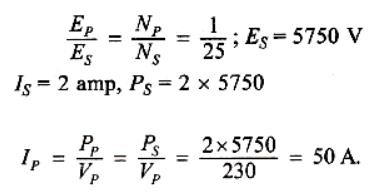QUESTION: 6

If the distance between the plates of parallel plate capacitor is halved and the dielectric constant of dielectric is doubled, then its capacity will

Solution: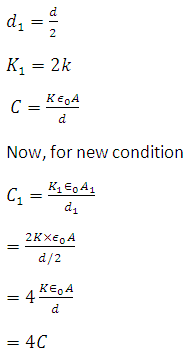QUESTION: 7

Two thin wire rings each having a radius R are placed at a distance d apart with their axes coinciding. The charges on the two rings are +q and -q. The potential difference between the centres of the two rings is

Solution: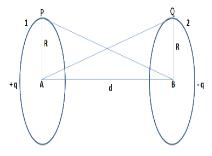Figure shows the two ring 1 and 2 each of radius R and having charges +q and −q
Let A and B be the centers of the two rings and VA and VB be the respective potentials at these points, then
VA= Potential due to ring 1+ Potential due to ring 2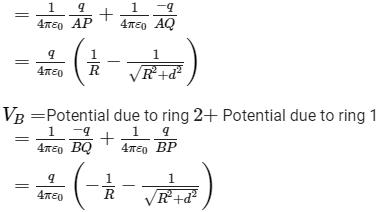Therefore potential difference between the two rings,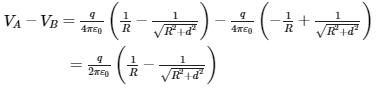QUESTION: 8

A mass of a gas exerts a pressure of 72 cm of mercury at 27o cm of mercury at 27oC. It is heated at constant volume and the pressure after some time becomes 90 cm of mercury. What is the temperature of the gas?

Solution:

(P1/T1)=(P2/T2)
T2=(P2.T1)/P1
T2=(90x300)/72
T2=375 K
T2=375-273 = 102oC

QUESTION: 9

The mass of the earth is 81 times that of the moon and the radius of earth is 3.5 times that of the moon. The ratio of the acceleration due to gravity at the surface of the moon to that at the surface of the earth is

Solution: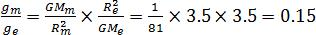QUESTION: 10

A particle moves in a circular orbit under the action of a central attractive force inversely proportional to the distance 'r'. The speed of the particle is

Solution:

We know that the centripetal force F = k/r
For some constant k and radius of curvature r.
Also we know centripetal force F = mv2 /r
Thus we get v = √k/m.

QUESTION: 11

A nucleus with Z = 92 emits the following in sequence: α , α , β , β , α , α , α , α ; β , β , α , β+ , β+ , α . The Z of the resulting nucleus is

Solution: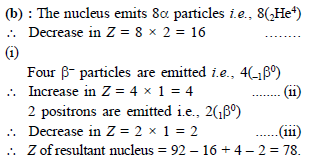QUESTION: 12

In the following question, a Statement of Assertion (A) is given followed by a corresponding Reason (R) just below it. Read the Statements carefully and mark the correct answer-
Assertion(A): Virtual images are always erect.
Reason (R): Virtual images are formed by diverging lenses only.

Solution:

The virtual image is always erect. The common example of virtual image is the image formed in the mirror when we stand in front of that mirror. A real image is that image which is formed when the light rays coming from an object actually meet each other after reflection or refraction.

QUESTION: 13

In the following question, a Statement of Assertion (A) is given followed by a corresponding Reason (R) just below it. Read the Statements carefully and mark the correct answer-
Assertion(A): According to law of conservation of mechanical energy sum of kinetic energy and potential energy is always constant.
Reason(R): Mechanical energy is conserve.

Solution:

Conservation of Mechanical Energy

The sum total of an object’s kinetic and potential energy at any given point in time is its total mechanical energy.  The law of conservation of energy says “Energy can neither be created nor be destroyed.”

So, it means, that, under a conservative force, the sum total of an object’s kinetic and potential energies remains constant.

QUESTION: 14

When seen in green light, the saffron and green portions of our National Flag will appear to be

Solution:

When seen in green light, the saffron and green portion of our national Flag will appear to be black and green respectively, because saffron absorbs the green light completely without any reflection so is shown as black, but Green portion of the Indian national flag reflects the same green light which falls on it so it appear as green in colour only.

QUESTION: 15

Which is not true; velocity of sound is

Solution:

The density of dry air is more than the moist air. When moisture is removed from air, its density increases. The speed of sound in a medium is inversely proportional to the square root of its density. Therefore, the speed of sound in moist air is more than that in dry air. Hence, correct answer is (D).

QUESTION: 16

Two tuning forks A and B produce 6 beats per second when sounded together. When B is slightly loaded with wax, the beats are reduced to 4 per second. If the frequency of A is 512 Hz, the frequency of 'B' is

Solution:

When loaded with wax, frequency of beats is reduced.
⇒ Frequency of B is higher than that of A.
⇒fB​−fA​=6
⇒fB​=(512+6)=518 Hz

QUESTION: 17

Heat capacity of a substance is infinite. It means

Solution:

Specific heat is defined as amount of joules to make 1 gram of a substance to change the temperature by 1 celsius; if the specific heat is infinite, that means it takes infinite amount of joules to change temperature, thus no change in temperature takes place even if you add or subtract heat because it would take infinite energy to change the temperature.

QUESTION: 18

The K.E. of a light and a heavy object is same Which object has more momentum?

Solution:
QUESTION: 19

In Young's double slit experiment, an interference pattern is obtained on a screen by a light of wavelength 6000 Å coming from the coherent sources S1 and S2. At certain point P on the screen third dark fringe is formed. Then the path difference S1p − S2p in microns is

Solution:

For dark fringe at P  S1p − S2p =Δ=(2n−1)λ/2
Here n =3 and l = 6000
So,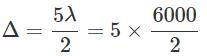=15000AA = 1.5 micron

QUESTION: 20

Two rods 1 and 2 are released from rest as shown in figure.
Given: l1=4l, m1=2m, l= 2l and m= ml1=4l, m1=2m,l2=2l and m2=m. There is no friction between the two rods. If αα be the angular acceleration of rod 1 just after the rods are released. Then :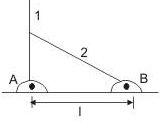Q. What is the normal reaction between the two rods at this instant ?

Solution:
QUESTION: 21

Two rods 1 and 2 are released from rest as shown in figure.
Given: l1=4l, m1=2m, l= 2l and m= ml1=4l, m1=2m,l2=2l and m2=m. There is no friction between the two rods. If αα be the angular acceleration of rod 1 just after the rods are released. Then :Q. What is the horizontal force on rod 1 by hinge A at this instant ?

Solution:
QUESTION: 22

Two rods 1 and 2 are released from rest as shown in figure.
Given: l= 4l, m= 2m, l= 2l and m= ml= 4l, m= 2m,l= 2l and m= m. There is no friction between the two rods. If αα be the angular acceleration of rod 1 just after the rods are released. Then :Q.
What is initial angular acceleration of rod 2 in terms of the given parameters in the question ?

Solution:
QUESTION: 23

In a series L-R circuit ,connected with a sinusoidal ac source, the maximum potential difference across L and R are respectively 3 volts and 4 volts.

Q. At an instant the potential difference across resistor is 2 volts. The potential difference in volts, across the inductor at the same instant will be:

Solution: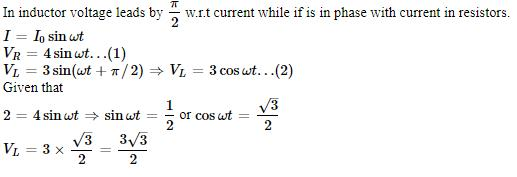QUESTION: 24

In a series L-R circuit ,connected with a sinusoidal ac source, the maximum potential difference across L and R are respectively 3 volts and 4 volts.

Q. At the same instant, the magnitude of the potential difference in volt, across the ac source will be

Solution:
QUESTION: 25

A block of base 10 cm x 10 cm and height 15 cm is kept on an inclined plane. The coefficient of friction between them is 3. The inclination θ of this inclined plane from the horizontal plane is gradually increased from 00 . Then

Solution:

As we increase the angle of inclination, the frictional force acts in upward direction on incline to prevent sliding of the block.
But this frictional force also causes torque about the CoM of the block, which is countered by the torque due to the Normal force on the block.
But as the angle of inclination θ increases, the normal force reduces and perpendicular distance of Normal force from CoM also reduces which decreases the counter-torque due to the normal force. So after an angle, the torque due to frictional force overcomes that due to normal force, and the block starts to topple.

QUESTION: 26

A fish looking through the water sees the world contained in a circular horizon. If the refractive index of water is 4/3 and the fish is 12 cm below the surface, what is the radius of the circle?

Solution:

Given that the refarctive index of water,μ = 4/3
The height between the fish and the surface of water, h = 12 cm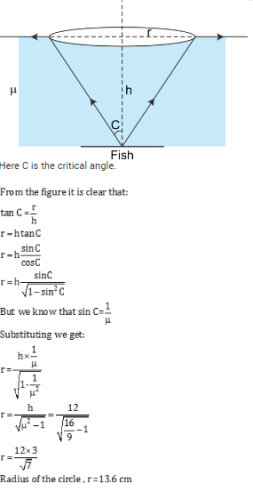QUESTION: 27

Two springs are connected to a block of mass M placed on a frictionless surface as shown in the fig. below. If both the springs have a spring constant k, the time period of oscillation of the block is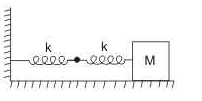Solution:

For spring block system,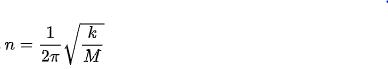Here two springs are joined in series so, total spring constant,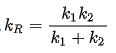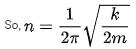so time period = 1/frequency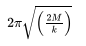QUESTION: 28

A body is released from a point at a distance r from the centre of the earth. Its velocity at the time of striking the earth (R is the radius of the earth and r>R) will be

Solution:
QUESTION: 29

A conducting wheel having 12 spokes out of which 7 are conducting and 5 are of non-conducting material is rotated with constant angular velocity ω about its own axis in a uniform magnetic field B whose direction coincides with that of the axis of wheel. The radius of wheel is R. The emf induced between the centre and periphery of the wheel is

Solution:
QUESTION: 30

A thin uniform rod of length l and mass m is swinging freely about a horizontal axis passing through its end. Its maximum angular speed is ω. Its centre of mass rises to a maximum height of-

Solution:

The uniform rod of length l and mass m is swinging about an axis passing through the end. When the centre of mass is raised through h, the increase in potential energy is mgh. This is equal to the kinetic energy.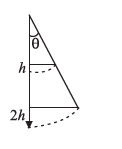= (1/2)Iω2.

⇒ mgh = 1/2(m(l2/3)).ω2

Therefore, h = (l2 . ω2)/6g.